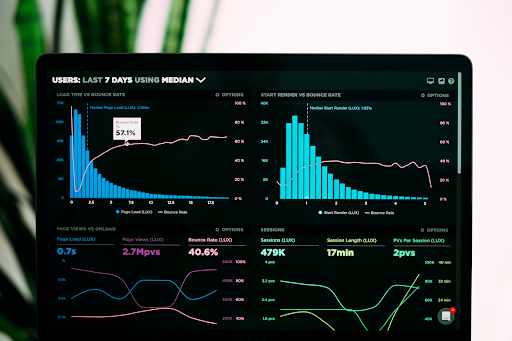# Creating Interactive Dashboards with Jupyter Notebooks and Plotly

Optimize your Jupyter Notebook performance using techniques such as upgrading to the latest version, lightweight libraries, lazy evaluation, parallel computing, GPU acceleration, efficient plotting, memory and code profilingAs a data scientist, you know the importance of presenting your findings in a clear and concise manner. One of the best ways to do this is through interactive dashboards, which allow users to explore data and draw their own conclusions. In this blog post, we’ll show you how to create interactive dashboards using Jupyter notebooks and Plotly. You can easily create these dashboards and more on Saturn Cloud.

## What is Jupyter Notebook?

Jupyter Notebook is an open-source web application that allows you to create and share documents that contain live code, equations, visualizations, and narrative text. It’s a popular tool among data scientists because it allows them to easily share their work with others and collaborate on projects.

## What is Plotly?

Plotly is a data visualization library that allows you to create interactive charts and graphs. It’s also open-source and can be used with a variety of programming languages, including Python, R, and MATLAB.

## Getting Started

To get started, you’ll need to install both Jupyter Notebook and Plotly. You can do this using pip, a package installer for Python.

``````\$ pip install jupyter
\$ pip install plotly
``````

Once you have both installed, you can start a new Jupyter Notebook by typing `jupyter notebook` in your terminal. This will open a new tab in your web browser where you can create a new notebook.

## Creating Interactive Charts with Plotly

To create an interactive chart with Plotly, you’ll first need to import the library and set up your data. For this example, we’ll use a simple dataset of car prices and mileage.

``````import plotly.express as px
import pandas as pd

fig = px.scatter(df, x='mileage', y='price', color='make')
fig.show()
``````

This code will create a scatter plot of car prices and mileage, with each point colored by the make of the car. You can hover over each point to see more information about the car, such as the make, model, and year.

## Adding Interactivity with Jupyter Widgets

While the chart is already interactive, we can add more interactivity using Jupyter widgets. Widgets are interactive controls that can be added to Jupyter notebooks, allowing users to manipulate data and see the results in real-time.

For this example, we’ll add a slider widget that allows users to filter the data by price. Here’s the updated code:

``````import ipywidgets as widgets
from IPython.display import display

price_slider = widgets.IntRangeSlider(
value=[df['price'].min(), df['price'].max()],
min=df['price'].min(),
max=df['price'].max(),
step=1000,
description='Price Range:',
layout={'width': '500px'}
)

def update_chart(prices):
filtered_data = df[(df['price'] >= prices) & (df['price'] <= prices)]
fig = px.scatter(filtered_data, x='mileage', y='price', color='make')
fig.show()

widgets.interact(update_chart, prices=price_slider)
``````

This code creates a slider widget that allows users to select a range of prices. The `update_chart` function is called every time the slider is changed, and it filters the data based on the selected price range. The updated chart is then displayed using Plotly.

By combining Jupyter Notebook and Plotly, you can create interactive dashboards that allow users to explore data and draw their own conclusions. In this blog post, we’ve shown you how to create a simple dashboard with a scatter plot and a slider widget. With these tools, you can create more complex dashboards that include multiple charts and widgets.